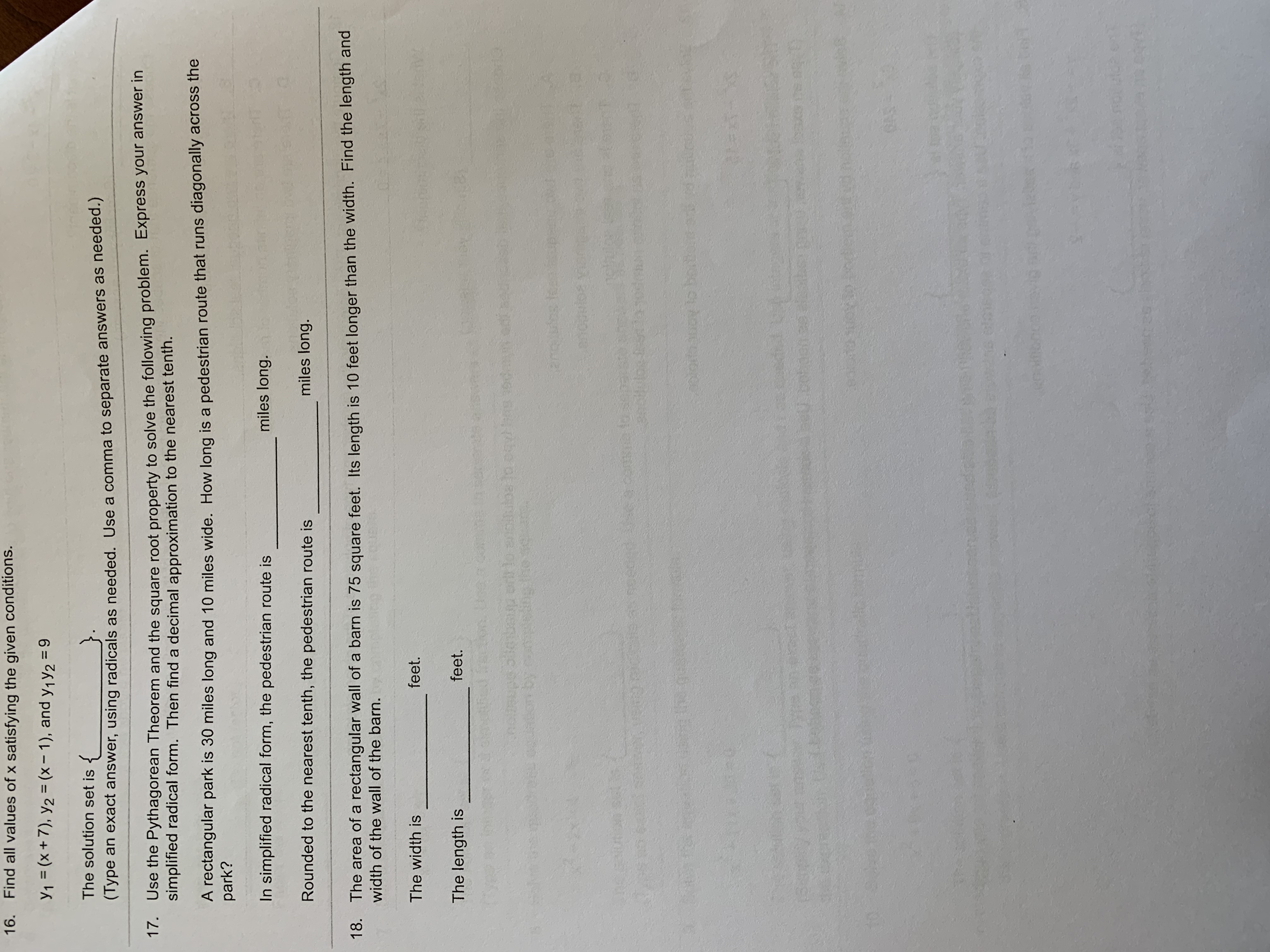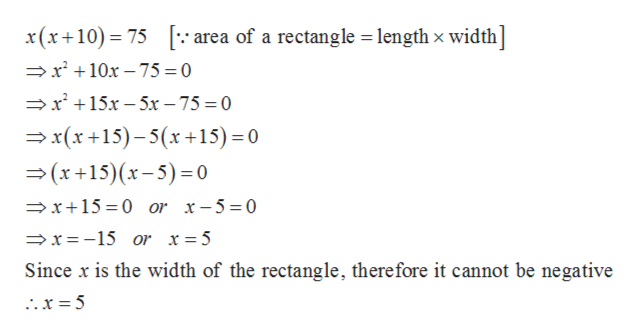# Find all values of x satisfying the given conditions.16.Y1 (x+7), y2 (x-1), and y1 y2 =9The solution set is(Type an exact answer, using radicals as needed. Use a comma to separate answers as needed.)17. Use the Pythagorean Theorem and the square root property to solve the following problem. Express your answer insimplified radical form. Then find a decimal approximation to the nearest tenth.A rectangular park is 30 miles long and 10 miles wide. How long is a pedestrian route that runs diagonally across thepark?miles long.In simplified radical form, the pedestrian route ismiles long.Rounded to the nearest tenth, the pedestrian route is18. The area of a rectangular wall of a barn is 75 square feet. Its length is 10 feet longer than the width. Find the length andwidth of the wall of the barn.feet.The width isfeet.The length isuealeba or lo iptuY Baong fsdareubentouoy tocotaon1.0YIC434

Question
4 views

Can someone help me solve this problem? #18help_outlineImage TranscriptioncloseFind all values of x satisfying the given conditions. 16. Y1 (x+7), y2 (x-1), and y1 y2 =9 The solution set is (Type an exact answer, using radicals as needed. Use a comma to separate answers as needed.) 17. Use the Pythagorean Theorem and the square root property to solve the following problem. Express your answer in simplified radical form. Then find a decimal approximation to the nearest tenth. A rectangular park is 30 miles long and 10 miles wide. How long is a pedestrian route that runs diagonally across the park? miles long. In simplified radical form, the pedestrian route is miles long. Rounded to the nearest tenth, the pedestrian route is 18. The area of a rectangular wall of a barn is 75 square feet. Its length is 10 feet longer than the width. Find the length and width of the wall of the barn. feet. The width is feet. The length is ue aleba or lo iptu Y Baong fsd areube nto uoy to cotaon 1.0YI C434 fullscreen
check_circle

Step 1

Given, the area of a rectangular wall of a barn is 75 square feet. its length is 10 feet longer than the width.

Step 2
Step 3

Now, area of the r...help_outlineImage Transcriptionclosearea of a rectangle length x width] x(x+10) 75 x10x-75 0 >r 15x-5x - 75 = 0 x(x +15)-5(x+15) = 0 (x+15) (x-5)= 0 x+15 0 or x-5 =0 x =-15 or x= 5 Since x is the width of the rectangle, therefore it cannot be negative fullscreen

### Want to see the full answer?

See Solution

#### Want to see this answer and more?

Solutions are written by subject experts who are available 24/7. Questions are typically answered within 1 hour.*

See Solution
*Response times may vary by subject and question.
Tagged in
MathAlgebra

### Equations and In-equations